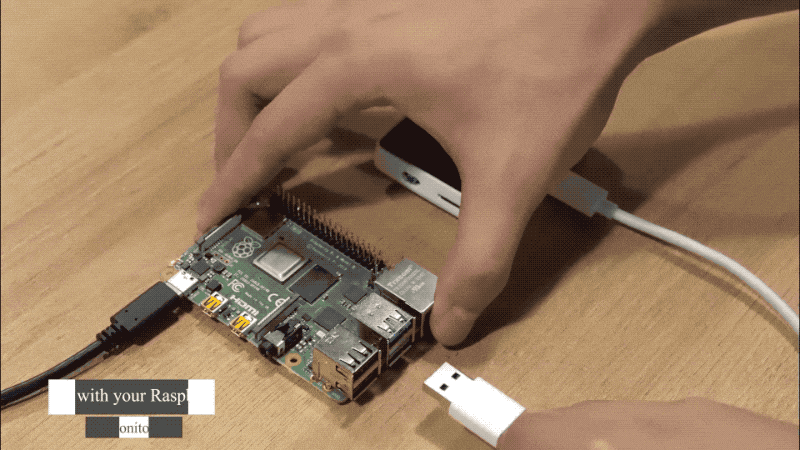edit

# Reading Raspberry Pi's System Status using Wio Terminal¶This wiki introduces how to read the system status of Raspberry Pi via USB serial communication using Wio Terminal. In this demo, the Raspberry Pi will be the host computer and sends its system information to the serial port via USB. Wio Terminal will be the slave and receives the incoming data and display on its LCD Screen.

## Features¶

• Reading Raspberry Pi's System status

• Display on Wio Terminal's Screen

## Raspberry Pi Host Program¶

Run the following Python code on Raspberry to send the system information to Wio Terminal via USB Serial.

Note: Please make sure that the Serial port is correct in the Python code, change it according to your device.

### Python Code on Raspberry Pi¶

import os
import time
import serial

# Settings for reading from Arduino Serial
serialPort= "/dev/ttyACM0" #Change it to your Serial Port, Check in Arudino IDE
baudRate = 115200
ser = serial.Serial(serialPort, baudRate, timeout=0.5)
time.sleep(2)

# Return CPU temperature as a character string
def getCPUtemperature():
return(res.replace("temp=","").replace("'C\n",""))

# Return RAM information (unit=kb) in a list
# Index 0: total RAM
# Index 1: used RAM
# Index 2: free RAM
def getRAMinfo():
p = os.popen('free')
i = 0
while 1:
i = i + 1
if i==2:
return(line.split()[1:4])

# Return % of CPU used by user as a character string
def getCPUuse():
return(str(os.popen("top -n1 | awk '/Cpu$$s$$:/ {print \$2}'").readline().strip()))

# Return information about disk space as a list (unit included)
# Index 0: total disk space
# Index 1: used disk space
# Index 2: remaining disk space
# Index 3: percentage of disk used
def getDiskSpace():
p = os.popen("df -h /")
i = 0
while 1:
i = i +1
if i==2:
return(line.split()[1:5])

def main():
while True:
# CPU informatiom
CPU_temp = getCPUtemperature()
CPU_usage = getCPUuse()

# RAM information
# Output is in kb, here I convert it in Mb for readability
RAM_stats = getRAMinfo()
RAM_total = str(round(int(RAM_stats) / 1000,1))
RAM_used = str(round(int(RAM_stats) / 1000,1))
RAM_free = str(round(int(RAM_stats) / 1000,1))

# Disk information
DISK_stats = getDiskSpace()
DISK_total = DISK_stats
DISK_used = DISK_stats
DISK_perc = DISK_stats

temp=ser.write(str.encode(CPU_temp+' '+CPU_usage))

data=ser.write(str.encode(CPU_temp+':'+CPU_usage+':'+RAM_total+':'+RAM_used+':'+RAM_free+':'+DISK_total+':'+DISK_used+':'+DISK_perc))
ser.flush()
time.sleep(2)

print('')
print('CPU Temperature = '+CPU_temp)
print('CPU Use = '+CPU_usage)
print('')
print('RAM Total = '+str(RAM_total)+' MB')
print('RAM Used = '+str(RAM_used)+' MB')
print('RAM Free = '+str(RAM_free)+' MB')
print('')
print('DISK Total Space = '+str(DISK_total)+'B')
print('DISK Used Space = '+str(DISK_used)+'B')
print('DISK Used Percentage = '+str(DISK_perc))

if __name__ == '__main__':
try:
main()
except KeyboardInterrupt:
if ser != None:
ser.close()


## Arduino Code¶

### Arduino libraries needed¶

• Install the LCD screen Library Seeed_Arduino_LCD, please visit Wio Terminal LCD for more information.

• Download the Free_Fonts.h header file so that can use abbreviations for the free fonts included in the LCD library. Please make sure to put this header file in the same location as the Arduino Sketch.

## Instructions¶

1. Upload the Arduino Code to Wio Terminal. Once uploaded it should be in a starting screen.

2. Connect Wio Terminal to the Raspberry Pi via USB Type-C Cable.

3. Run the Python code on Raspberry Pi.

4. Now, you should be able to see the Raspberry's Pi System info on Wio Terminal's LCD Screen.

## Tech Support¶

Please submit any technical issue into our forum.